Cramér theorem

An integral limit theorem for the probability of large deviations of sums of independent random variables. Let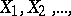be a sequence of independent random variables with the same non-degenerate distribution function, such that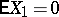and such that the generating functionof the moments is finite in some interval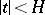(this last condition is known as the Cramér condition). Let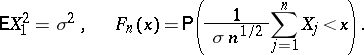If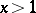,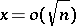as, then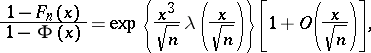Here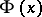is the normal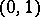distribution function andis the so-called Cramér series, the coefficients of which depend only on the moments of the random variable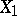; this series is convergent for all sufficiently small. Actually, the original result, obtained by H. Cramér in 1938, was somewhat weaker than that just described.